Electron. J. Diff. Equ., Vol. 2014 (2014), No. 93, pp. 1-14.

### Existence and multiplicity of positive periodic solutions for second-order functional differential equations with infinite delay Qiang Li, Yongxiang Li

Abstract:
In this article, the existence and multiplicity results of positive periodic solutions are obtained for the second-order functional differential equation with infinite delay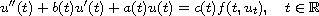whereare continuous-periodic functions,is defined by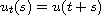for,denotes the Banach space of bounded continuous function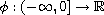with the norm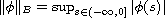, and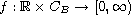is a nonnegative continuous functional. The existence conditions concern with the first eigenvalue of the associated linear periodic boundary problem. Our discussion is based on the fixed point index theory in cones.

Submitted September 22, 2013. Published April 4, 2014.
Math Subject Classifications: 34C25, 47H10.
Key Words: Second-order functional differential equations; first eigenvalue; positive periodic solution; cone; fixed point index.

Show me the PDF file (272 KB), TEX file, and other files for this article.Qiang Li Department of Mathematics Northwest Normal University Lanzhou 730070, China email: lznwnuliqiang@126.com Yongxiang Li Department of Mathematics Northwest Normal University Lanzhou 730070, China email: liyxnwnu@163.com, Phone 86-0931-7971111